# Lesson 8: Modeling Population Growth

Populations -- whether human, animal, bacterial, etc -- all have common dynamics. Although there are variations, the unifying theme is the appearance of exponential functions. We will develop the theory behind exponential population growth and analyze it several different ways.

# The Mathematical Model(s)

### Model 1: Synchronized Generations

Let's start simple and work our way up. We'll try to model a growing population of bacteria, which satisfies the following assumptions (a biologist might tell you these are questionable, but they're roughly correct):

1. Every so often, the bacteria split in two.
2. This division happens to every single cell.
3. This division is perfectly synchronized: it happens to all cells simultaneously.
4. The length of time between divisions (a "generation") is always the same.
5. There are no other factors (space, disease, predators) influencing the population.

Let's convert the assumptions into a symbolic equation, which we can then work with. First we need some symbols. Let's usefor time, measured in generations, and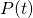to denote the population in generation.

Since each bacterium splits into two, after a division there are twice as many bacteria as there were before. So we have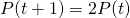Assumptions 1 and 2 are the "2" in the equation; assumptions 3 and 4 are the fact that no matter whatis, we know the population 1 generation later; assumption 5 is the fact that there are no other terms involved in determining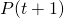except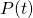itself.

This is another finite difference model, like the ones we considered in Lessons 6-I and 6-II. So we can use Excel to explore it iteratively. Let's say the colony starts off with just a single bacterium in the zeroth generation.(Unlike in Lessons 5, 6, and 7, where we had several parameters to play with, here we've built the parameters into our model: they are "2", and the fact that we are working in "generations".)

Dragging down in column B reveals:after 46 generations, there are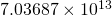bacteria in the colony. For some perspective, that's about 200 times the number of stars in the Milky Way. Since these are bacteria, it could well be that a "generation" only takes, say, an hour. So in less than 2 days we go from 1 bacterium to more bacteria than there are stars in the sky, and then some. Makes you want to go wash your hands, doesn't it?

In Excel, the notation 7.89E 6 means "7.89 times 10 to the sixth power", or 7.89 million. 7.89E-6 means "7.89 times 10 to the negative-sixth power", or 7.89 millionths. This has nothing to do with the number e that we use as the base for the exponential function.

Actually, what the absurd largeness of this number reveals is that our model is probably not correct. So let's refine it.

### Model 2: Bacteria Take Turns, Still Synchronized

In practice, not everyone who's eligible to reproduce actually does. Maybe a particular bacterium is hungry, or cold, or tired, or stressed from work and doesn't feel like it tonight. Some bacteria will reproduce; some won't. Let's assume that

• the fraction of cells which divide in a given generation is constant across generations

Then our finite difference model needs to be updated. The cells in generation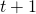are of two kinds: either they were those who didn't divide from generation, or they're descendants of cells who did divide in generation. As a word equation: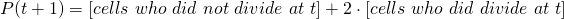If we letrepresent the fraction of cells which divide in each generation, then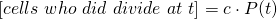and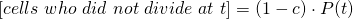.

Putting it all together, we obtain the new finite difference model: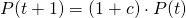(I skipped some algebra -- either work it out yourself, or have Maple do it for you, like I demonstrate here.)

Let's see what Excel says about this if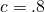, i.e. if we assume that 80% of bacterial cells in our colony divide when they can:After 46 generations, the population is "only"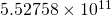-- roughly equal to the number of stars in the Milky Way (still a bit more).One observation about both Model 1 and Model 2 is that it's easy to solve them analytically by hand. What I mean is: since the finite difference equation is so simple, we just substitute to find: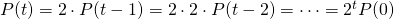in Model 1, or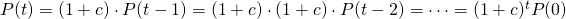in Model 2.

Here the pattern was easier to find than in Lesson 6-I and Lesson 6-II, but we could have had Maple do it, too:Observe that these are both exponential functions: they involve a constant (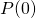) times another constant (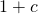) raised to the power of the independent variable.

### Model 3: Synchronized Generations Are Really Weird, Y'all!

To me, the weirdest assumption that went into Models 1 and 2 is that the bacteria be reproducing according to a synchronized schedule. For bacteria, maybe that's not quite as weird. But for people, think about how odd that would be. (Some cultures do have big orgiastic festivals during spring, which means that births end up being synchronized in the following winter.) You'd a whole bunch of babies, and a whole bunch of 22-year-olds, but nobody in between (also, nobody would be allowed to choose to delay having a child till later, or to have one earlier).

To fix this, we need to move away from discrete "generations" and into continuous time. Let's replace our assumption from model 2 with:

• Across any interval of time, for any given bacterium, there is a chance that bacterium divides. This chance is proportional to the length of the time interval, but otherwise does not depend on time.

We can rewrite this in the following way: consider a time interval fromto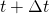. Our assumption is that the chance that the bacterium will divide in this interval is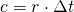, for some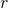. Just as we did in Model 2, we have: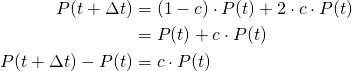And if we divide through by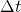, we obtain: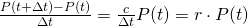and now we letgo to zero (i.e. let our discrete time interval get shorter and shorter, like we did in Lesson 7), to obtain the differential equation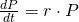What functionsolves this equation? Let's ask Maple!That's Maple's way of saying that a general solution to the population model should be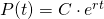. Which is also an exponential function!

Now, let's see how to interpret the parameters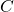and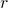.is what we get when we plug in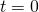, so it represents the initial population.

What about? Remember, to interpret a parameter, think about units. If we are counting bacteria and measuring time in hours, the units of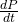are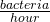. So in order forto be true, the units ofmust be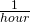. The way to understand this is:measures the hourly rate of reproduction of the bacteria. If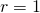, then bacteria are reproducing at a rate of 100% per hour; if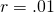, then they are reproducing at 1% per hour.

Let's apply this model to another population: the population of brown rats in New York City. We'll work with time denominated in years. Let's say that on average, each female rat gives birth to a 5 litters each year, with each litter consisting of 8 baby rats, 4 of which are female. Then, at least approximately, each female rat will produce 20 female rats each year. Let's say that only 10 of these survive to sexual maturity. (We're only counting female rats for obvious reasons.) So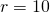, give or take. Let's say we start with a total population of 4 rats, 2 male and 2 female.  How many rats are there after six months (half a year)? To get Maple to answer this, we use dsolve and subs:Almost every program (Excel, Maple, Mathematica, you name it) will react in unexpected ways if you type "e^10". Instead, get in the habit of writing "exp(10)".

So that's about 297 female rats after six months, descended from the original 2; in total there should be twice that many, or 594, rats.

# Takeaways/Deliverables

To say you've successfully completed this lesson, you should be able to do the following:

1. Derive a finite-difference model for constant-rate reproduction.
2. Use Maple's dsolve to solve an initial value problem.
3. Use Maple's subs and evalf to evaluate values of exponential functions.

These skills are what you'll need to complete the WebAssign homework for this Lesson.